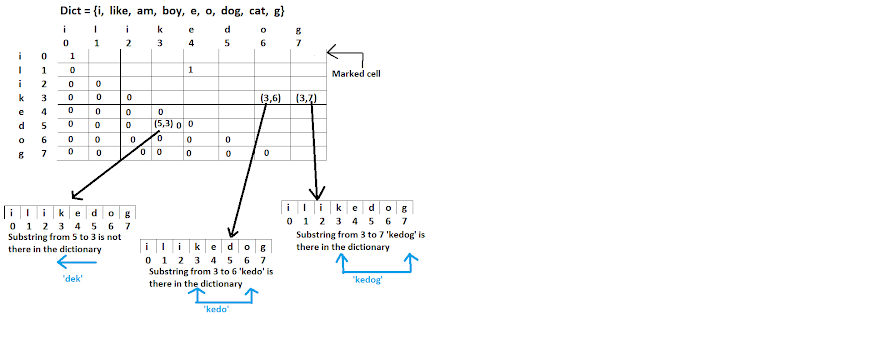# How to solve word break problem in Java using dynamic programming? Example

Problem Statement:
You are given a dictionary of words and the input string. Determine input string can be segmented into a space-separated sequence of given dictionary words.

Note: This question is based on dynamic programming and asked multiple times in top product-based companies.

Inputs:

Dict = {i, like, am, boy, e, o, dog, cat, g};

word = "iIikedog"  --------can be segmented into space-separated words--------> i, like, dog

Ask yourself that 'I', 'like', and 'dog' are presented in the dictionary?

Yes, so we can say that the given string can be segmented.

Now let's take another example.

word = "ilikecatsanddog" --------- can be segmented as-----> i, like, cats, and, dog

i, like, cat, s, and, dog

We can see that the words 'cats', 'and', 's' are not present in the dictionary so such a string can't be segmented into space-separated words.

Let's try to solve it.....

Like another dynamic programming problem, we will create a matrix and will use previously calculated results to calculate the current result.

Consider the given string as an array like this.

 i l i k e d o g

The columns and rows represent the same given string in the matrix.Now let's try to understand what each cell represents in the matrix.

The cells can have two kinds of values either '0' or '1'. Suppose the cells (3,6) and (3,7) have the value '1' this means the substrings from 3 to 6 and 3 to 7 are present in the given input dictionary.

If you notice the cell (5,3) and the corresponding substring in the given word the direction is reverse and there is no point in considering the reverse computation of the given string. So we will mark all such cells as '0'.

We filled half of the matrix with value '0' so we no need to perform the reverse computations for these cells. This way we can save both memory and time.

Now start filling rest of the cells manually by comparing the row-column pair value against the given dictionary.

So the cell (0, 0) i.e. 'i' is present in the dictionary so matrix = 1.

Similarly cell (4, 4) i.e. 'e' is also present in the dictionary so matrix = 1.

Now let's take one substring 'ilike' and see the logic to solve this problem.

 i l i k e d o g 0 1 2 3 4 5 6 7

i      l     i     k      e

0     1    2    3      4

We will try to separate this string into 2 parts in all the possible ways.

Let say we have first 2 parts:

(0, 0)--------> 'i' and (1, 1)----------> 'l'

matrix && matrix

1 && 0

So 'il' is not present in the dictionary.

Consider another combination.

(4, 3)--------> 'k' and (4, 4)----------> 'e'

matrix && matrix

0 && 1

This is false means 'ke' is not present in the dictionary.

So I will keep on taking the substrings from the given word to be space separated and divide them into 2 parts in different ways and will check against the matrix that if both the parts are true i.e. '1' means the substring is present in the dictionary.

So finally we will consider the entire given word and divide it into 2 parts in all the possible ways and we will use the values of the previously computed cells to calculate the value of the marked cell and if the the value is '1' means the given word can be separated into the space separated segments.

Start Implementing it...

Consider below code snippet.

 i l i k e d o g 0 1 2 3 4 5 6 7

For i = 0 and N = 7

for(int k = 0; k < N+1; k++){

if(matrix[i][i+k]) && matrix[k+1][N])

{

return true;

}

}

The if condition shows the logic to divide the given word into 2 parts in all possible ways.

Complete Code:

public class Main {

public String wordBreakProblem(String word, Set<String> dict){

int matrix[][] = new int[word.length()][word.length()];

// fill all the cells with '-1'.

for(int i=0; i < matrix.length; i++){

for(int j=0; j < matrix[i].length ; j++){

matrix[i][j] = -1;

}

}

//If the substring is present in the dictionary then fill the corresponding cell with non-negative value.

for(int l = 1; l <= word.length(); l++){

for(int i=0; i < word.length() -l + 1 ; i++){

int j = i + l-1;

String str = word.substring(i,j+1);

if(dict.contains(str)){

matrix[i][j] = i;

continue;

}

// Filling the value of the corresponding cell for the taken substring using value of the previously calculated cell.

for(int k=i+1; k <= j; k++){

if(matrix[i][k-1] != -1 && matrix[k][j] != -1){

matrix[i][j] = k;

break;

}

}

}

}

if(matrix[word.length()-1] == -1){

return null;

}

//Finally segregate the given word into the words available in the dictionary.

StringBuffer buffer = new StringBuffer();

int i = 0; int j = word.length() -1;

while(i < j){

int k = matrix[i][j];

if(i == k){

buffer.append(word.substring(i, j+1));

break;

}

buffer.append(word.substring(i,k) + " ");

i = k;

}

return buffer.toString();

}

public static void main(String args[]){

Set<String> dictionary = new HashSet<String>();

Main bmw = new Main();

String result1 = bmw.wordBreakProblem(str, dictionary);

System.out.print(result1);

}

}

Output:

Q. 1) Given dict = {'li, 'sop', 'tree', 'ding', 'g'} and word = 'sopptreeg'. Is it possible to segment the given string into space separated segments of the given dict. words ?

Ans. 'sop' , 'p', 'tree', 'g'

'so', 'pp', 'tree', 'g'

It looks like the given word can't be separated.

Before you leave...

Knowledge of data structure and algorithms is must to simulate the real world problem in code.